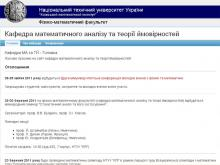﻿

Department of Mathematical Analysis and Probability Theory (MATAN), FMF

﻿The Higher Mathematics Department №1 was transformed into Mathematical Analysis and Probability Theory Departmen due to the formation of Faculty of Physics and Mathematics in 1996 and it was made the graduating department. Mathematical Analysis and Probability Theory department has 48 highly qualified lecturers including 5 professors and 22 associate professors.

From 1986 to 2012 the head of the department was Honored Worker of Science of Ukraine (2009), Laureate of the State Prize of Ukraine (2003), Doctor of Physical and Mathematical Sciences, Professor Buldygin Valeriy Volodymyrovich. Since 2012 the head of the department is Doctor of Physical and Mathematical Sciences, professor Klesov Oleg Ivanovich. The workers of the Department are lecture the following courses to the 1st-6st year students of Faculty of Physics and Mathematics, Department of Aircraft and Space Systems, Faculty of Electronics, Faculty of Informatics and Computer Science, Faculty of Management and Marketing, Intercollegiate Faculty of Medical Engineering, Faculty of Radio Engineering, Military Institute of Telecommunications and Information Technology, Institute for Applied Systems Analysis, Institute of special communication and information Security, Institute of Telecommunication Systems:

1. Higher Mathematics
2. Mathematical Analysis
3. Linear Algebra and Analytic Geometry
4. Discrete Mathematics
5. Complex Analysis
6. Probability Theory and Mathematical Statistics
7. Theory of measure and integral
8. Functional Analysis
9. Introduction to Stochastic Processes Theory
10. Theory of Stochastic Processes
11. Operators Theory
12. Statistics of Random Processes
13. ntegral transformations
14. Markov processes
15. Additional chapters of mathematical analysis
16. Special chapters of mathematics
17. Methods of problem solving in higher mathematics
18. Statistical Analysis
19. Stochastic analysis and its application

The department graduates specialists and masters in mathematics. The graduates are allocated to work at the universities, scientific research institutes, schools, insurance companies, banks, etc. The best of them stay to work in the Department and to take a post-graduate school in specialties:

• 01.01.01 -- Mathematical Analysis
• 01.01.05 -- Probability Theory and Mathematical Statistics
x

Департамент навчальної роботи

Розклад занять Розклад сесії Розклад для викладачів
x

Медіа-ресурси КПІ

Web-камери Радіо Відеорепортажі КПІ